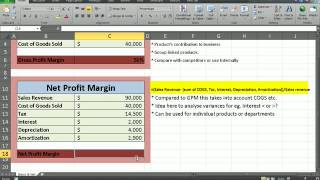# net profit

Este contenido está disponible en los siguientes idiomas: Español | Inglés

NET PROFIT = Total Revenue - Total Expenses.

Also known as after tax benefit: After tax benefits are the profits (or money) left to a company after an operating cycle by subtracting the cost of goods sold, the operating cost, interest and taxes.

It can be calculated with the earnings and losses account. Calculating this value is necessary to get the net operating margin, which is simply the ratio between the net operating profit and sales.

# MultimediaFinancial Analysis- Calculating net and gross profit using excel